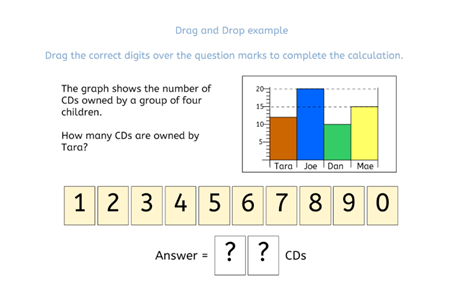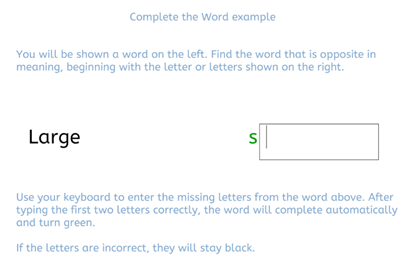# Entrance Examination

Pupils for the Entrance Exam will sit three tests: a one-hour computer-based test, and two half-hour written papers. Collectively, these tests will focus on pupils’ verbal, non-verbal and mathematical ability, as well as their ability to write accurately and creatively.

#### 1. One Computer-Based Test

This test is one hour long, or 1 hour and 15 minutes for pupils with extra time. Pupils will complete the test on devices provided by the school. The test has several timed sections that cover verbal ability (vocabulary, grammar and comprehension), numerical ability (arithmetic and problem-solving), and non-verbal ability. This test evaluates the academic potential of candidates rather than emphasising their prior knowledge.

Although the test is designed not to require excessive preparation, we encourage all candidates to look at the following website prior to the entrance examination: https://plus.cem.org/cemselectfam/.

Question types will include the following:

• Multiple choice: click on one of several options available. The option selected will be highlighted in yellow. Here is an example question:• Drag and drop: drag one or more of the options into the boxes or areas available. Here is an example question:• Complete a word: using the keyboard of the device provided, type in the letters required to complete a word. After entering two correct letters, the word will automatically complete. Here is an example question:#### 2. Two Paper-Based Tests

In addition to the computer-based test outlined above, candidates for the Entrance Examination will sit an English paper of half an hour followed by a Mathematics paper of half an hour. Pupils with extra time will have 37.5 minutes per paper, meaning a total of one hour and fifteen minutes.

For the half-hour English paper, candidates will be asked to produce a piece of creative writing in response to a prompt such as a picture or title. For example, pupils might be asked to produce a piece of writing that describes a particular setting, such as a jungle. Candidates will be assessed on the quality of their writing, including the following:

• The accuracy of their writing, including their spelling of complex words, their grammar and their punctuation

• The ambition of their vocabulary, including well-used complex words

• Their ability to write in a way that is suitable to the given purpose, for example using sentence length and structure to create a mood of excitement

• Their ability to make use of a variety of sentence structures, including simple, compound and complex sentences

• Their ability to use paragraphs accurately and effectively

• The creativity and originality of their writing

You can find a Sample English Paper at the bottom of this page.

For the half-hour Mathematics paper, candidates are advised familiarise themselves with the following Topic List:

Whole Number Arithmetic

• Adding and subtracting two, three or four digit numbers

• Multiplying and dividing numbers - traditional methods preferred but no need for long division.

• Multiplying and dividing by powers of 10

• Be familiar with the terms odd, even, prime, multiple, factor

Decimal Fraction Arithmetic

• Understand place value

• Adding and subtracting decimal numbers

• Multiplying and dividing by powers of 10

Vulgar Fraction Arithmetic

• Work out percentage ‘of’ a quantity

• Be able to compare the sizes of fractions, percentages and decimals

• Be able to find the fraction of a shape that is shaded

Geometry

• Know that amount of turn can be measured in degrees

• Know that angles on a line sum to 180 degrees

• Know that angles in a triangle sum to 180 degrees

• Know that the angles around a point sum to 360 degrees

• Know how to find the area of a square, rectangle and compound shapes involving rectangles

• Know how to find the area of a triangle

• Know the units of area

• Be able to recognise the following shapes: Isosceles triangle, equilateral triangle, trapezium, kite

• Know and be able to use the following words: parallel, right angle, acute angle, obtuse angle, reflex angle, perpendicular.

• Be able to plot points and read coordinates in the first quadrant

• Be able to identify the order of rotational symmetry of a shape

• Be able to identify lines of symmetry

Measure

• Be able to make calculations involving time 12 and 24h

• Know metric units of length and conversion factors

Probability and Statistics

• Be able to find a probability for equally likely events

• Be able to interpret and draw bar graphs

• Be able to interpret pie charts

• Be able to find a mode and mean